Home > A2C > Chapter 11 > Lesson 11.2.1 > Problem11-41

11-41.
1. Solve each equation below for x. Show all of your work. (Your answers will contain the variables a, b, and/or c.) Homework Help ✎

1. cx − a = b

2.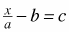3. (xa)(xb) = 0

4. ax 2acx = 0

5.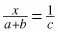6.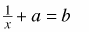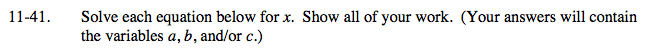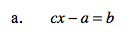$x = \frac{a + b}{c}$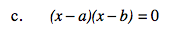x = a or x = b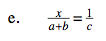Multiply both sides by c(a + b).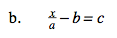x = ab + ac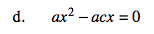Factor and use the Zero Product Property.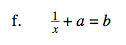Subtract a from both sides and take the reciprocal of both sides.